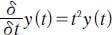NextPrevious

# What is an example of a differential equation?

An example of a differential equation involves letting y be some function of the independent variable t. Then a differential equation relating y to one or more of its derivatives is as follows:In this equation, the first derivative of the function y is equal to the product of t2 and the function y itself. This also implies that the stated relationship holds only for all t for which both the function and its first derivative are defined.

Close

This is a web preview of the "The Handy Math Answer Book" app. Many features only work on your mobile device. If you like what you see, we hope you will consider buying. Get the App